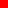Home | Science | Gravitation | * Gravitation 3.8 Home Page — Normal Display Gravitation 3.8 Home Page — Big Display Gravitation 3.8 Home Page — Small Display Gravitation Help/TutorialShare This Page
Gravitation Help/TutorialThis page explains the theory behind gravitational modeling, and offers some useful hints for using the Gravitation program.Helpful Hints

• Choose a scenario and, before running it, move one or more of the bodies around with your mouse — this makes the orbits change completely, sometimes the "planets" crash into each other, lots of interesting things happen.
• To make the simulation as fast as possible, go to the "Setup" menu and click "Highest Computer Speed." This won't work on some browsers.
• To speed up the simulation's progress through time, increase the value of "Time Interval." This will decrease the accuracy of the simulation but speed it up.
• Conversely, to increase the accuracy of your simulation at the expense of running time, decrease the value of "Time Interval."
• To see some nice patterns, choose the "Three Mutual Orbit" or "Four Mutual Orbit" scenarios using the drop-down list and select "Lines" for the display mode.
• Instead of moving the bodies with your mouse, you can go to the "Data" menu and type in new values for position, velocity and radius. The new radius value automatically changes the mass of the body, so this changes the orbital behavior as well as the body's size on the screen.
• While running a simulation, switch to the "Data" menu to see the numbers change. The data menu also helps in deciding whether a "planet" has escaped your screen's universe or will be coming back eventually.
• You can select a scenario and then use the "Data" menu to create a completely new scenario of your own.
• Gravitation can be run as a "Stand-alone" Java application. Simply place all the provided .CLASS files in a convenient directory and use your Java resources to launch the program.The Theory and Strategies Behind "Gravitation"

The basic gravitational equation is:where F equals the force between two masses M1 and M2, R is the radius (distance) between the two masses, and G, the universal gravitational constant, is equal to 6.670 x 10-8 dyne cm2 gm-2. This formula gives the force between two masses at a particular instant of time. This formula is based on the work of Isaac Newton and is the basic equation in what is called "Newtonian Gravitation," gravitation in which relativistic effects are not taken into account.

To model the behavior of masses across time, things get a bit more complicated. If there are only two masses being modeled, then a relatively simple set of equations can be used. If more than two masses are involved, one must apply the above formula repeatedly, apply the resulting accelerations to the masses, and repeat the process in a computer simulation. This is what "Gravitation" does.

Numerical gravitation simulations are never more than approximations of the behavior of masses in nature. The limitations are:

1. One must select a finite time interval for each iteration of the program. If the chosen interval is small, this increases accuracy but requires more computer time. If the chosen interval is large, this speeds up the simulation but at the cost of decreased accuracy.
2. The floating point numbers used in computers have limited resolution. This resolution limit can degrade the accuracy of the simulation over many iterations.

The effect of these limitations can be dramatically seen in the "Three Mutual Orbit" scenario, where the three bodies gradually begin to lose their original orientation, perform an intricate dance, and finally fly apart at great speed. Some of this behavior can be traced to limitations in the numerical simulation and do not represent real gravitational effects. The rest of the scenario is a valid representation of nature — three mutually attracting bodies of equal masses tend to be unstable.

In some of Gravitation's scenarios, two bodies will approach and then fly apart at high speed. This is a limitation of numerical gravity simulation, it is not a property of natural gravitation — if it were, it would violate the principle of energy conservation. It comes about because a particular body may pass very close to another, experience that body's gravitational attraction, then on the next iteration be located far past that same body, so there is no deceleration to match the acceleration, as there would be in nature. This effect can be minimized by choosing small increments of time, but it cannot be eliminated. This incremental effect, and the errors brought on by computer floating point numbers, are limitations in all numerical gravitational simulations, not just the one performed by Gravitation.

The method used in Gravitation is the same as that used in large research facilities to, for example, model the evolution of star clusters. The computation time required to model a set of N mutually interacting bodies is (more or less) proportional to N2, because each body's gravitational acceleration is the sum of all the other bodies' influences. Therefore, for each body in the system, the program must calculate the sum of the accelerations of all the other bodies. This computational difficulty explains why stellar cluster modeling is an important application for supercomputers.These Pages Created and Maintained usingArachnophilia.### Main Page

 Home | Science | Gravitation | * Gravitation 3.8 Home Page — Normal Display Gravitation 3.8 Home Page — Big Display Gravitation 3.8 Home Page — Small Display Gravitation Help/TutorialShare This Page